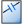APhotoconductive Semiconductor Switch (PCSS) circuit component definition simulates a semiconductor device that conducts electricity when illuminated.

PCSS components should be used when only time-domain results are of interest. This is because PCSS components violate the assumptions of linear system theory, making it incorrect to apply either a Fourier or discrete Fourier transform to the time-domain results. A simulation containing a PCSS produces invalid frequency-domain results—including impedances, S-parameters, and steady-state far-zone fields—even if the time-domain results eventually decay to zero. The time-domain results are unaffected, and therefore are valid.

XF's PCSS component is not illuminated at the beginning of a simulation and is equivalent to a passive resistor, as expressed in the following equation:

At a user-defined illumination time the resistance's rate of change is determined based on the value of $V_s$. When $V_s \gt 0$, the transition follows a smooth curve determined by

\begin{equation} \frac{\dot R}{R} = \frac{1}{T_r}-\frac{\text{tanh}\left(\frac{|V|-V_a}{V_s}\right)+1}{2T_a} \qquad \text{when} \qquad t \geq T_i, V_s\gt 0 \end{equation}

If $V_s=0$, then the rate of change is abrupt and follows the piecewise fuctions

\begin{eqnarray} \frac{\dot R}{R} &=& \frac{1}{T_r}-\frac{1}{T_a}&\qquad\text{when}\qquad t \geq T_i, V_s= 0, |V|\gt V_a \\ \frac{\dot R}{R} &=& \frac{1}{T_r}&\qquad\text{when}\qquad t \geq T_i, V_s= 0, |V| \lt V_a \end{eqnarray}

where

$R =$ instantaneous cell edge resistance

$\dot R/R =$ time rate of change of $R$

$R_0 =$ initial resistance

$V =$ instantaneous cell edge voltage

$V_a =$ avalanche voltage

$V_s =$ normalized inverse rate of change of $1/T_a$ with voltage

$T_i =$ illumination time

$T_a =$ exponential avalanche carrier generation time

$T_r =$ exponential carrier recombination time

$R$ is capped at $R_0$ in the illuminated state because it would otherwise tend to infinity at low $V$, such as at the end of a simulation.Double-clicking on a circuit component definition in the Definitions branch of the Project Tree opens the Circuit Component Definition Editor, where a PCSS is created by choosing Photoconductive Semiconductor Switch from the Type drop-down menu.

Rated Voltage & Current settings apply to electrostatic discharge (ESD) analysis. Both Rated Voltage and Rated Current default to infinity, but users can change either value by unchecking the associated box and entering the desired value or expression. These settings do not affect energy propagation through the component, but XF checks the voltage and current at each timestep during a simulation to see if they exceed the rated values. Users can adjust these settings to flag components that are likely to experience damage or failure during a simulation.